Home > CC4 > Chapter 11 > Lesson 11.2.5 > Problem11-110

11-110.
1. Solve the equations and inequalities below, if possible. Homework Help ✎

2.  a. 6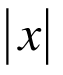> 18 b.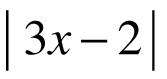≤ 2 c. (4x − 2)2 = 100 d. (x − 1)3 = 8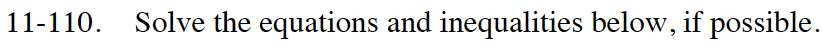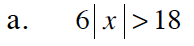x < −3 or x > 3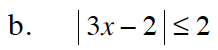Begin by changing the inequality to an equation and solve for the boundary points.

$\left|3x-2\right|=2$

Since |±2| = 2, this can be rewritten as two equations. Solve the two equations for x and plot the boundary points on a number line.
3x − 2 = 2 3x − 2 = −2

Test points on the number line in the original inequality to find the regions that make it true. Shade them and describe them algebraically.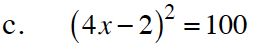4x − 2 = 10 4x − 2 = −10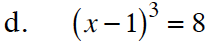Look inside. The cube root of 8 is 2.